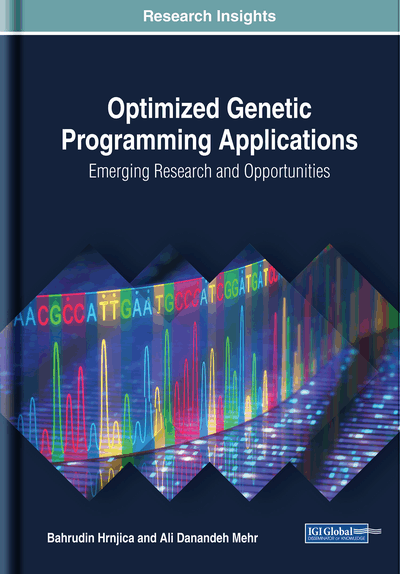# Different Approaches in Genetic Programming

DOI: 10.4018/978-1-5225-6005-0.ch003

## Abstract

The GP method explained in previous chapters was about the evolution of computer programs represented by monolithic gene (syntax tree). This is the original and most widespread type of GP that is also referred to as tree-based GP. In recent years, new variants of GP have emerged that follow the basic idea of traditional GP to automatically evolve computer programs, but the programs are evolved/represented in different ways. New variants of GP include but are not limited to stack-based genetic programming, linear genetic programming (LGP), Cartesian genetic programming, grammatical evolution (GE), graph-based GP (GGP), context-free grammar (CFGGP), multigene genetic programming (MGGP), and gene expression programming (GEP). Among these variants, main features, evolution of computer programs, and a brief review of engineering applications of MGGP, GEP, and LGP are introduced in this chapter.
Chapter Preview
Top

## Multigene Genetic Programming (Mggp)

MGGP is a variant of GP that linearly combines low-depth GP trees in order to improve fitness of the potential solutions of GP. Owing to the use of smaller trees, MGGP is expected to provide simpler models than those of standard GP (Searson 2015). In MGGP, the output variable is computed by the summation of weighted outputs of two or more GP trees in a multigene program plus a stochastic term, so-called bias. For example, the pseudo-linear MGGP model shown in Figure 1 represents the output variableas a combination of three standard GP trees (Gene 1, Gene 2, and Gene 3) comprising two input variables (x1 and x2). Mathematically, this model can be written as Equation (1).

(1)

## Complete Chapter List

Search this Book:
Reset